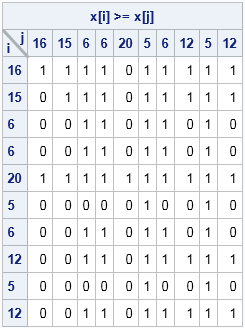A SAS customer showed me a SAS/IML program that he had obtained from a book. The program was taking a long time to run on his data, which was somewhat large. He was wondering if I could identify any inefficiencies in the program.

The first thing I did was to "profile" the program to identify trouble spots. (See Ch. 15, "Timing Computations and the Performance of Algorithms," in Statistical Programming with SAS/IML Software.) I discovered one function that was taking about 80% of the total time. The inefficient function (if I may oversimplify the problem) essentially took a vector x with N elements and returned an N x N indicator matrix, A, whose (i, j)th element is 1 if x[i] ≥ x[i] and is 0 otherwise. A small example of the x vector is used in the following statements. The inefficient code looked something like this:

```proc iml; x = {16, 15, 6, 6, 20, 5, 6, 12, 5, 12}; /* data for pairwise comparison */ N = nrow(x);   A = j(N,N,0); do i = 1 to N; do j = 1 to N; if x[i] >= x[j] then A[i,j] = 1; /* A[i,j] = 1 if x[i] >= x[j]; 0 otherwise */ end; end;```The 10 x 10 indicator matrix for this example is shown. When N is large, this double DO loop approach can be slow. I have written about similar computations before. For example, I've written about how to compute pairwise differences for a vector of values, which is almost the same problem. The key is to eliminate nested DO loops and replace them with vector or matrix operations, a process known as vectorization.

### Pairwise comparisons: An exercise in vectorization

To implement a vector-based computation, think about the jth column of A. What is the jth column? It is the indicator vector obtained by comparing x to the jth value of x. The following statements rewrite the computation by using a single DO loop that iterates over each column of the resulting matrix:

```B = j(N,N,0); do j = 1 to N; B[,j] = (x >= x[j]); end;```

Equivalently, you could loop over rows of the indicator matrix by using B[i,] = (x` <= x[i]) (but notice the direction of the inequality!). Either method produces a big speedup in the computation.

A matrix approach is less intuitive, but can be more efficient unless the matrices are huge. The idea is to form a matrix with N columns, each column being a copy of the data vector x. If you compare the elements of this matrix to the elements of its transpose, you obtain the desired indicator matrix:

```xx = repeat(x, 1, N); /* the i_th row is x[i] */ C = (xx >= xx`); /* indicator matrix */```

The matrices A, B, and C are equal.

### A slight twist

In the previous section, I oversimplified the problem. The real problem evaluated several complicated logical expressions (by using IF-THEN statements) within the doubly-nested DO loops. The result of those logical expressions affected the values of the indicator matrix. To simplify matters, I'll illustrate the situation by using a binary vector and a simple logical AND operator. Assume that the IF-THEN statements resolve to either 1 or 0 (true or false) for each value of the column index, j, as follows:

```s = {0, 1, 1, 0, 0, 1, 0, 1, 1, 0}; /* result of logical expressions, j=1 to N */   A = j(N,N,0); do i = 1 to N; do j = 1 to N; if x[i] >= x[j] & s[j]=1 then /* more complicated IF-THEN logic: */ A[i,j] = 1; /* A[i,j] = 1 if x[i] >= x[j] AND s[j]=1 */ end; end;```

Although this new situation is more complicated, the lessons of the previous section remain relevant. Here's how you can compute the indicator matrix by using vector operations:

```B = j(N,N,0); do j = 1 to N; B[,j] = (x >= x[j]) & (s[j]=1); end;```

Here's the equivalent matrix operations:

```xx = repeat(x, 1, N); ss = repeat(s, 1, N); C = (xx >= xx`) & (ss`=1);```

If the logic within the doubly-nested loops depends on both rows and columns (i and j), then the variable s becomes a matrix and the computations are modified accordingly.

In summary, many SAS/IML programmers know that looping over rows and columns of a matrix is inefficient and that vectorizing the computations leads to performance improvements. This article applies the same principles to pairwise comparisons of data in a vector. By avoiding scalar comparisons, you can improve the performance of the computation.

Share